Test: Frequency Response

# Test: Frequency Response

Test Description

## 10 Questions MCQ Test Topicwise Question Bank for Electronics Engineering | Test: Frequency Response

Test: Frequency Response for Electronics and Communication Engineering (ECE) 2022 is part of Topicwise Question Bank for Electronics Engineering preparation. The Test: Frequency Response questions and answers have been prepared according to the Electronics and Communication Engineering (ECE) exam syllabus.The Test: Frequency Response MCQs are made for Electronics and Communication Engineering (ECE) 2022 Exam. Find important definitions, questions, notes, meanings, examples, exercises, MCQs and online tests for Test: Frequency Response below.
Solutions of Test: Frequency Response questions in English are available as part of our Topicwise Question Bank for Electronics Engineering for Electronics and Communication Engineering (ECE) & Test: Frequency Response solutions in Hindi for Topicwise Question Bank for Electronics Engineering course. Download more important topics, notes, lectures and mock test series for Electronics and Communication Engineering (ECE) Exam by signing up for free. Attempt Test: Frequency Response | 10 questions in 30 minutes | Mock test for Electronics and Communication Engineering (ECE) preparation | Free important questions MCQ to study Topicwise Question Bank for Electronics Engineering for Electronics and Communication Engineering (ECE) Exam | Download free PDF with solutions
 1 Crore+ students have signed up on EduRev. Have you?
Test: Frequency Response - Question 1

### The mid frequency gain of the amplifier circuit shown below with high value of beta of the BJT is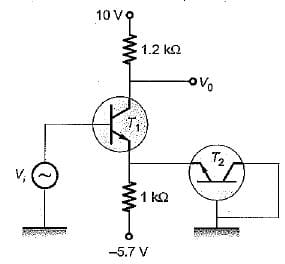Detailed Solution for Test: Frequency Response - Question 1

Applying KVL, we get: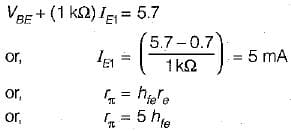Now,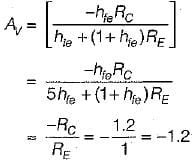or, this diagram is CE unbypass amplifier so,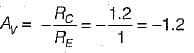Test: Frequency Response - Question 2

### In a common source MOSFET amplifier circuit operating at 10 kH z, the drain resistance Rd = 200 kΩ. If MOSFET parameters are gm = 1.5 mA/V, r d = 50 kQ, Cds = 1.0 pF and Cgd = 2.0 pF, the voltage gain is equal to

Test: Frequency Response - Question 3

### An npn BJT has gm = 38 mΩ/V, Cμ = 10-14 F, Cπ = 4 x 10-13 F and DC current gain, β0 = 90. For this transistor fT and fβ are respectively

Detailed Solution for Test: Frequency Response - Question 3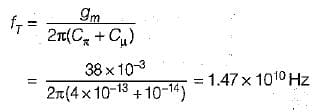and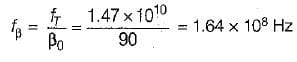Test: Frequency Response - Question 4

G iven for an FET, gm = - 9 .5 m A /volt, total capacitance = 500 pF. For a voltage gain of -30, the bandwidth will be

Test: Frequency Response - Question 5

The drain gate capacitance of a junction FET is 2 pF. Assuming a common source voltage gain of 20, what is the input capacitance due to miller effect?

Test: Frequency Response - Question 6

Assertion (A): In the high frequency model of FET, the voltage amplification drops drastically with increase in frequency.
Reason (R): The high frequency model is identical with the low frequency model except that the capacitances between various terminals (gate, source and drain) is added.

Detailed Solution for Test: Frequency Response - Question 6

The high frequency mode! of FET is shown below.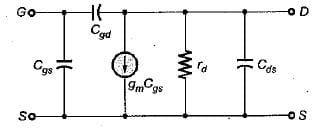Because of the internal capacitances (Cgd, Cgs and Cds), feedback exists between the output and input circuits of the FET in its high frequency model and hence the volta g e amplification drops drastically with the increase in frequency. Moreover, there is no feedback from output to input in the FET small signal (low frequency) model.
Flence, both assertion and reason are correct and reason is the correct explanation of assertion.

Test: Frequency Response - Question 7

For a single stage CE amplifier, gm = 50 mA/V, Ce= 1 pF and Cc = 0.2 pF. The frequency at which the short circuit common-emitter current gain attains unit magnitude is given by

Detailed Solution for Test: Frequency Response - Question 7

The frequency at which the short circuit common- emitter current gain attains unit magnitude is given by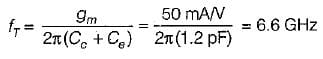Test: Frequency Response - Question 8

When the load resistance is zero (short circuit) in a common-emitter configuration, the current flowing from the base to the collector node through the collector capacitor Cc, is small because

Detailed Solution for Test: Frequency Response - Question 8

Ce can be as smai| as 1 pF, and Cc as small as 0.2 pF.
Thus, Cis larger than Cc

Test: Frequency Response - Question 9

Consider the following statements:
1. For finding the transistor behaviour at high frequency it is necessary to examine the diffusion mechanism.
2. At low frequency it is assumed that the transistor responds instantly to changes of input voltage or current.
3. The hybrid-jr model is valid under dynamic conditions when the rate of change of \/BEis small enough so that the base incremental current/^ is small compared with the collector incremental current/^
4. The parameter fTis a frequency characteristic of a transistor.

Which of the above statements are correct?

Detailed Solution for Test: Frequency Response - Question 9
• The mechanism of the transport of charge carriers from emitter to collector is essentially one of diffusion. Hence, for finding the transistor behaviour at high frequency it is n e ce ssa ry to exam ine the d iffu sio n mechanism. Hence, statement-1 is correct.
• Statement-2 is also correct.
• Statement-3 is also correct.
• The frequency at which the short-circuit common-emitter current gain attains unit magnitude is represented by fT Thus, Statement - 4 is also correct.
Test: Frequency Response - Question 10

A modern bipolar transistor can have Ce = 1 pF. If gm = 50 mA/V, the fT of a common emitter amplifier will be

Detailed Solution for Test: Frequency Response - Question 10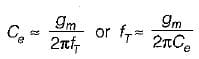or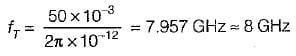## Topicwise Question Bank for Electronics Engineering

101 tests
 Use Code STAYHOME200 and get INR 200 additional OFF Use Coupon Code
Information about Test: Frequency Response Page
In this test you can find the Exam questions for Test: Frequency Response solved & explained in the simplest way possible. Besides giving Questions and answers for Test: Frequency Response, EduRev gives you an ample number of Online tests for practice

101 tests